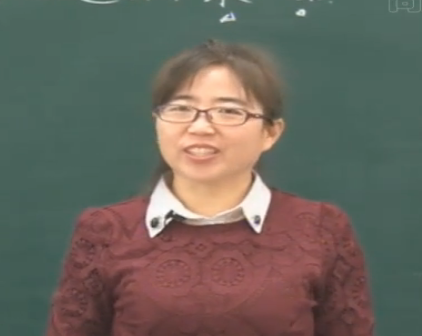## 尊敬的用户：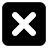### 三年级数学上册第三章《加与减》捐书活动—三位数连加

• 同步课程
• 课后作业
• 同步试卷
• 本节重点
• 主讲老师

197+268=  405+219=
678+85=   327+69=

118比103多15，
104比109少5，
95比98少3...

118+104+95=（）

100+100+100=300
18+4-5=17
300+17=317

100+100=200
10+90=100
8+4+5=17
200+100+17=317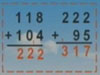118+104+95
=222+95
=317（本）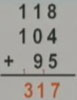118+104+95=317（本）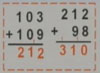103+109+98
=212+98
=310（本）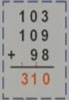103+109+98=310（本）

127+114+86    297+304+120
175+260+149   292+450+108

（1）相同数位要对齐；
（2）符号要写准确；
（3）标好进位，在计算时一定要加进去。

## 一、填空题

1．试一试，写出下面每个图形表示的数。☆=
□=
△=

【试题解析】：解决这类数字谜问题，需要从个位入手：3个△相加的和不可能是5，说明此处有进位，也就是：3×△=15，推算出△是5；十位上□+5+5加上进位的1结果不可能是1，说明此处仍有进位，也就是□+5+5+1=11，推算出□是0；百位上☆+☆+5+1=20，推算出☆是7。
【规律方法】：

【参考答案】：7、0、5

## 二、主观题

1．圈一圈。
(1)哪三个星星上的数相加最接近300?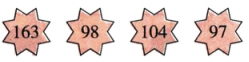(2)哪两个星星上的数相加最接近400?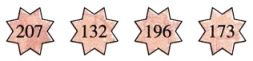【试题解析】：
【规律方法】：

【参考答案】：(1)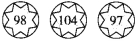(2)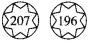2．用竖式计算下面各题。
128+67+308=     135+75+588=     447+366+187=

【试题解析】：
【规律方法】：

【参考答案】：503  798  1000（竖式略）

3．买家具。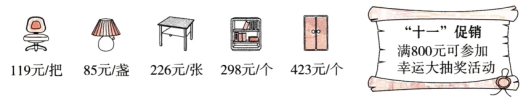(1)收银员应收李阿姨多少元？李阿姨可以参加幸运大抽奖活动吗？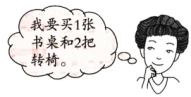(2)估一估，如果想参加幸运大抽奖活动，可以买哪3件不同的家具？（写出两种不同的方案）

【试题解析】：
【规律方法】：

【参考答案】：(1)226+119+119=464（元）    464元＜800元

(2)423+298+226≈950（元）
423+298+119≈840（元）